### The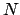-adic Numbers

Lemma 16.2.5   Letbe a positive integer. Then for any nonzero rational number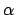there exists a unique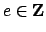and integers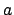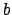, withpositive, such that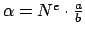with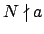,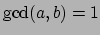, and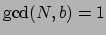.

Proof. Write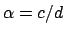with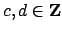and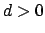. First suppose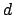is exactly divisible by a power of, so for some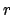we have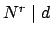but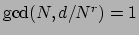. Then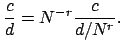If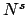is the largest power ofthat divides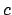, then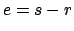,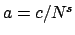,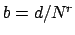satisfy the conclusion of the lemma.

By unique factorization of integers, there is a smallest multiple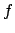ofsuch that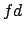is exactly divisible by. Now apply the above argument withandreplaced by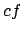and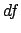.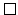Definition 16.2.6 (-adic valuation)   Letbe a positive integer. For any positive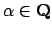, the ofis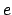, whereis as in Lemma 16.2.5. The-adic valuation of 0 is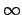.

We denote the-adic valuation ofby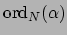. (Note: Here we are using valuation'' in a different way than in the rest of the text. This valuation is not an absolute value, but the logarithm of one.)

Definition 16.2.7 (-adic metric)   For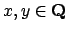the between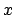and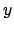is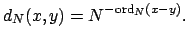We let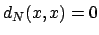, since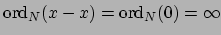.

For example,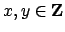are close in the-adic metric if their difference is divisible by a large power of. E.g., if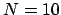then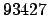and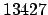are close because their difference is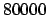, which is divisible by a large power of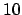.

Proposition 16.2.8   The distance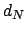on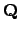defined above is a metric. Moreover, for all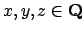we have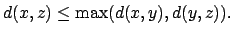(This is the nonarchimedean'' triangle inequality.)

Proof. The first two properties of Definition 16.2.1 are immediate. For the third, we first prove that if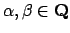then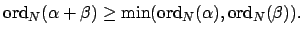Assume, without loss, that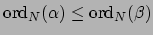and that bothand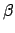are nonzero. Using Lemma 16.2.5 write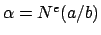and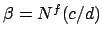withorpossibly negative. Then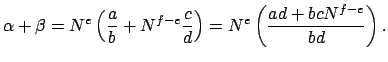Since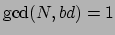it follows that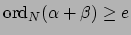. Now suppose. Then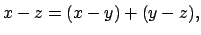so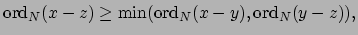hence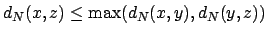.We can finally define the-adic numbers.

Definition 16.2.9 (The-adic Numbers)   The set of , denoted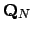, is the completion ofwith respect to the metric.

The setis a ring, but it need not be a field as you will show in Exercises 57 and 58. It is a field if and only ifis prime. Also,has a bizarre'' topology, as we will see in Section 16.2.3.

William Stein 2004-05-06